# sql语句的执行顺序:

1. FROM <left_table>
2. ON <join_condition>
3. <join_type> JOIN <right_table>
4. WHERE <where_condition>
5. GROUP BY <group_by_list>
6. HAVING <having_condition>
7. SELECT
8. DISTINCT <select_list>
9. ORDER BY <order_by_condition>
10. LIMIT <limit_number>

1. LIMIT 语句

SELECT *
FROM   operation
WHERE  type = 'SQLStats'
AND name = 'SlowLog'
ORDER  BY create_time
LIMIT  1000, 10;

SELECT   *
FROM     operation
WHERE    type = 'SQLStats'
AND      name = 'SlowLog'
AND      create_time > '2017-03-16 14:00:00'
ORDER BY create_time limit 10;

2. 隐式转换

SQL语句中查询变量和字段定义类型不匹配是另一个常见的错误。比如下面的语句：

mysql> explain extended SELECT *
> FROM   my_balance b
> WHERE  b.bpn = 14000000123
>       AND b.isverified IS NULL ;
mysql> show warnings;

| Warning | 1739 | Cannot use ref access on index 'bpn' due to type or collation conversion on field 'bpn'

3. 关联更新、删除

UPDATE operation o
SET    status = 'applying'
WHERE  o.id IN (SELECT id
FROM   (SELECT o.id,
o.status
FROM   operation o
WHERE  o.group = 123
AND o.status NOT IN ( 'done' )
ORDER  BY o.parent,
o.id
LIMIT  1) t);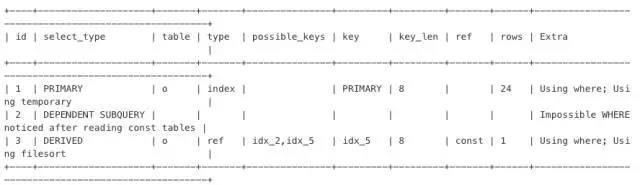UPDATE operation o
JOIN  (SELECT o.id,
o.status
FROM   operation o
WHERE  o.group = 123
AND o.status NOT IN ( 'done' )
ORDER  BY o.parent,
o.id
LIMIT  1) t
ON o.id = t.id
SET    status = 'applying'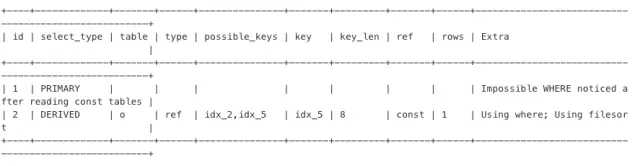4. 混合排序

MySQL 不能利用索引进行混合排序。但在某些场景，还是有机会使用特殊方法提升性能的。

SELECT *
FROM   my_order o
INNER JOIN my_appraise a ON a.orderid = o.id
a.appraise_time DESC
LIMIT  0, 20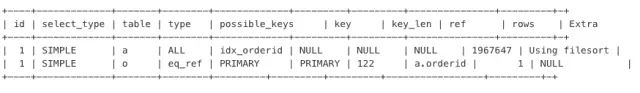SELECT *
FROM   ((SELECT *
FROM   my_order o
INNER JOIN my_appraise a
ON a.orderid = o.id
ORDER  BY appraise_time DESC
LIMIT  0, 20)
UNION ALL
(SELECT *
FROM   my_order o
INNER JOIN my_appraise a
ON a.orderid = o.id
ORDER  BY appraise_time DESC
LIMIT  0, 20)) t
appraisetime DESC
LIMIT  20;

5. EXISTS语句

MySQL 对待 EXISTS 子句时，仍然采用嵌套子查询的执行方式。如下面的 SQL 语句：

SELECT *
FROM   my_neighbor n
LEFT JOIN my_neighbor_apply sra
ON n.id = sra.neighbor_id
AND sra.user_id = 'xxx'
WHERE  n.topic_status < 4
AND EXISTS(SELECT 1
FROM   message_info m
WHERE  n.id = m.neighbor_id
AND m.inuser = 'xxx')
AND n.topic_type <> 5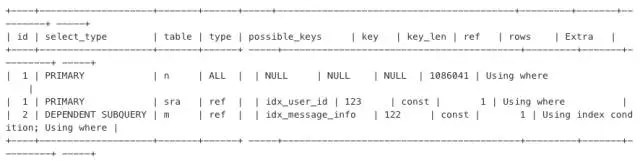SELECT *
FROM   my_neighbor n
INNER JOIN message_info m
ON n.id = m.neighbor_id
AND m.inuser = 'xxx'
LEFT JOIN my_neighbor_apply sra
ON n.id = sra.neighbor_id
AND sra.user_id = 'xxx'
WHERE  n.topic_status < 4
AND n.topic_type <> 5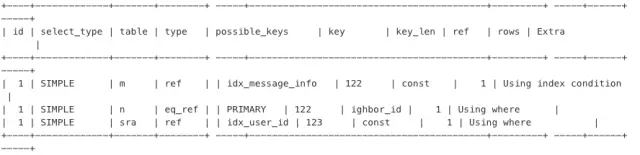6. 条件下推

UNION 或 UNION ALL 子查询；

SELECT *
FROM   (SELECT target,
Count(*)
FROM   operation
GROUP  BY target) t
WHERE  target = 'rm-xxxx'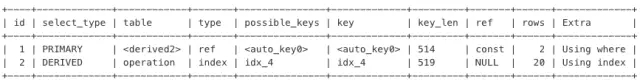SELECT target,
Count(*)
FROM   operation
WHERE  target = 'rm-xxxx'
GROUP  BY target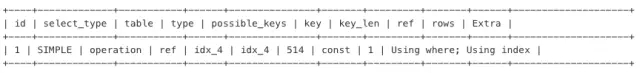7. 提前缩小范围

SELECT *
FROM   my_order o
LEFT JOIN my_userinfo u
ON o.uid = u.uid
LEFT JOIN my_productinfo p
ON o.pid = p.pid
WHERE  ( o.display = 0 )
AND ( o.ostaus = 1 )
ORDER  BY o.selltime DESC
LIMIT  0, 15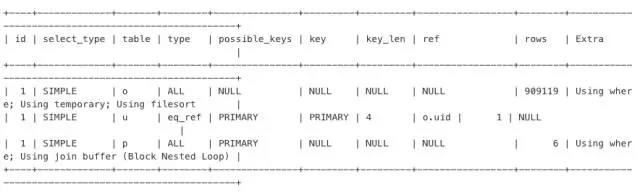SELECT *
FROM (
SELECT *
FROM   my_order o
WHERE  ( o.display = 0 )
AND ( o.ostaus = 1 )
ORDER  BY o.selltime DESC
LIMIT  0, 15
) o
LEFT JOIN my_userinfo u
ON o.uid = u.uid
LEFT JOIN my_productinfo p
ON o.pid = p.pid
ORDER BY  o.selltime DESC
limit 0, 15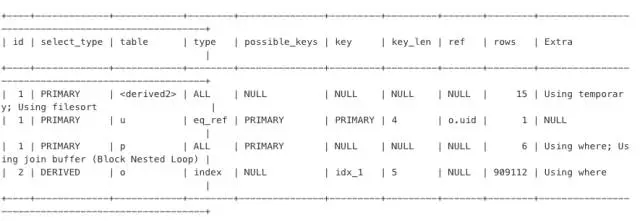8. 中间结果集下推

SELECT    a.*,
c.allocated
FROM      (
SELECT   resourceid
FROM     my_distribute d
WHERE    isdelete = 0
AND      cusmanagercode = '1234567'
ORDER BY salecode limit 20) a
LEFT JOIN
(
SELECT   resourcesid， sum(ifnull(allocation, 0) * 12345) allocated
FROM     my_resources
GROUP BY resourcesid) c
ON        a.resourceid = c.resourcesid

SELECT    a.*,
c.allocated
FROM      (
SELECT   resourceid
FROM     my_distribute d
WHERE    isdelete = 0
AND      cusmanagercode = '1234567'
ORDER BY salecode limit 20) a
LEFT JOIN
(
SELECT   resourcesid， sum(ifnull(allocation, 0) * 12345) allocated
FROM     my_resources r,
(
SELECT   resourceid
FROM     my_distribute d
WHERE    isdelete = 0
AND      cusmanagercode = '1234567'
ORDER BY salecode limit 20) a
WHERE    r.resourcesid = a.resourcesid
GROUP BY resourcesid) c
ON        a.resourceid = c.resourcesid

WITH a AS

SELECT   resourceid
FROM     my_distribute d
WHERE    isdelete = 0
AND      cusmanagercode = '1234567'
ORDER BY salecode limit 20)
SELECT    a.*,
c.allocated
FROM      a
LEFT JOIN
(
SELECT   resourcesid， sum(ifnull(allocation, 0) * 12345) allocated
FROM     my_resources r,
a
WHERE    r.resourcesid = a.resourcesid
GROUP BY resourcesid) c
ON        a.resourceid = c.resourcesid©️2019 CSDN 皮肤主题: 技术黑板 设计师: CSDN官方博客### 分享到微信朋友圈点击重新获取扫码支付